# Non Verbal Reasoning - Pattern Completion

### Exercise :: Pattern Completion - Section 2

In each of the following questions, select a figure from amongst the four alternatives, which when placed in the blank space of figure (X) would complete the pattern.

11.

Identify the figure that completes the pattern.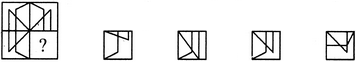(X)                 (1)             (2)              (3)             (4)

 A. 1 B. 2 C. 3 D. 4

Answer: Option C

Explanation: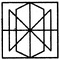12.

Identify the figure that completes the pattern.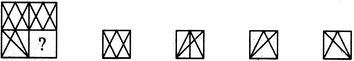(X)                 (1)             (2)              (3)             (4)

 A. 1 B. 2 C. 3 D. 4

Answer: Option C

Explanation: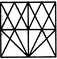13.

Identify the figure that completes the pattern.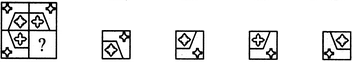(X)                 (1)             (2)              (3)             (4)

 A. 1 B. 2 C. 3 D. 4

Answer: Option B

Explanation:14.

Identify the figure that completes the pattern.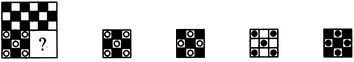(X)                 (1)             (2)              (3)             (4)

 A. 1 B. 2 C. 3 D. 4

Answer: Option B

Explanation: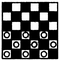15.

Identify the figure that completes the pattern.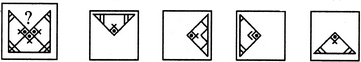(X)                 (1)             (2)              (3)             (4)

 A. 1 B. 2 C. 3 D. 4

Answer: Option D

Explanation:#### Current Affairs 2021

Interview Questions and Answers